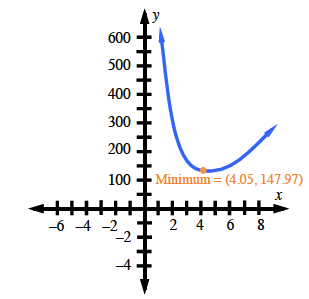### Home > A2C > Chapter 14 > Lesson 14.9.1.3 > Problem9-39

9-39.

The manufacturing company you work for has been hired to produce a $100$ cubic foot box. It must have a square base and no top. The material for the base costs $3$ per square foot and the material for the sides costs $1$ per square foot. We want to write an equation for the cost of the box as a function of $x$, the length of one edge of the base.

1. Write an expression for the cost of the base in terms of $x$.

Write and expression for the area and multiply that by the cost per square foot.

Area: $x^{2}$
Cost: $3x^{2}$

2. Express the cost of the sides in terms of $x$ and $h$, the height of the box.

Write an expression for the area of one side.
Write an expression for the area of four sides.
Write an expression for the cost of four sides.

Area: $4xh$
Cost: $\left(1\right)\left(4xh\right) = 4xh$

3. Express the total cost of the box in terms of $x$ and $h$.

This is the sum of your expressions from parts (a) and (b).

4. Use the fact that the volume of the box is $100$ cubic feet to eliminate h from the equation you just wrote and express the cost solely in terms of $x$.

$100 = x^{2}h$

$h = \frac{100}{x^2}$

Substitute 'h' into the equation in part (c).

5. Graph the equation and find the value of $x$ which gives the minimum cost. What is the minimum cost?